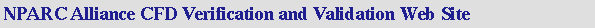V&V Home       Archive       Tutorial

## Verification Assessment

This page discusses Verification Assessment, which focuses on the methods for Verification of CFD codes and simulation results.

### Verification

Verification is defined as:

The process of determining that a model implementation accurately represents the developer's conceptual description of the model and the solution to the model. (AIAA G-077-1998)

Verification assessment examines 1) if the computational models are the correct implementation of the conceptual models, and 2) if the resulting code can be properly used for an analysis. The strategy is to identify and quantify the errors in the model implementation and the solution. The two aspects of verification are the verification of a code and the verification of a calculation. The objective of verifying a code is error evaluation, that is, finding and removing errors in the code. The objective of verifying a calculation is error estimation, that is determining the accuracy of a calculation. Each are discussed below.

Verification has also been described as solving the equations right. It is intended to concern itself more with mathematics rather than engineering. It is intended to look for errors in the programming and implementation of the models.

Roache considers two aspects of verification: 1) verification of a code and 2) verification of a calculation. These two aspects are described below:

Verification of a code involves error evaluation, which is, looking for bugs, incorrect implementations of conceptual models, errors in inputs, and other errors in the code and usage. This is typically done by the developers prior to release of the code. First, consistency checks are performed which examine basic relationships expected in the solutions (i.e. mass conservation). Then the code is used to simulate a suite of ``highly accurate'' verification cases. These cases should be analytic or numeric solution to ordinary and partial differential equations. Verification should not performed with experimental data. A grid refinement study should be conducted to bring out potential errors. All the options of the code should be examined. This becomes more complicated as the number of options available within a CFD code increase. Identifying and quantifying each type of error is important because errors can interact and cancel each other - leading to erroneous conclusions in the validation process. One potentially useful method of verification is comparing the results of two codes. However, verification is not a democratic activity and one should watch for comparing with an inaccurate code. The comparison is strengthened when the two codes use differing numerical methods. The following paragraphs discuss specific checks that can be performed as part of a code verification process.
Verification of a calculation involves error estimation, which is determining the accuracy of a single calculation and putting an error band on the final value. The approach involves peformimg a grid convergence study and determine the observed order of convergence, error bands, and grid convergence indices (GCI).

### Verification Assessment Process

The process for Verification Assessment of a CFD code and / or simulation can be summarized as:

1. Examine the Computer Programming of the Code.

One of the most basic tasks of verification assessment is the review of the computer programming or coding to check for and identify computer programming errors or "bugs". This is done by visually checking the coding and by computationally running subprograms using a test code. This is aided by complete and clear documentation, both internal and external. This step is to directly detect computer programming errors.

2. Examine Iterative Convergence.

Verification assessment requires that a simulation demonstrates iterative convergence. Further details can be page entitled Examining Iterative Convergence.

3. Examine Consistency.

One should check for consistency in the CFD solution. For example, the flow in a duct should maintain mass conservation through the duct. Further total pressure recovery in an inlet should stay constant or decrease through the duct.

4. Examine Spatial (Grid) Convergence.

The CFD simulation results should demonstrate spatial convergence. Further details and methods can be found on the page entitled Examining Spatial (Grid) Convergence.

5. Examine Temporal Convergence.

The CFD simulation results should demonstrate temporal convergence. Further details and methods can be found on the page entitled Examining Temporal Convergence.

6. Compare CFD Results to Highly Accurate Solutions

The veracity of a code can be examined by comparing the CFD simulation results to highly accurate solution to the models used within the CFD code. This can include analytical solutions, benchmark numerical solutions to ordinary differential equations (ODEs), and benchmark numerical solutions to partial differential equations (PDEs).
Last Updated: Thursday, 17-Jul-2008 09:56:53 EDT